# Create & run a basic PLSPM Project in Excel 2003

2017-03-03

This tutorial will show you how to create and run a basic Partial Least Squares Path Modeling (PLS-PM) project in Excel 2003 using the XLSTAT software.

## Principles of PLS path modeling

Partial Least Squares Path Modeling (PLS-PM) is a statistical approach for modeling complex multivariable relationships (structural equation models) among observed and latent variables. Since a few years, this approach has been enjoying increasing popularity in several sciences (Esposito Vinzi et al., 2007). Structural Equation Models include a number of statistical methodologies allowing the estimation of a causal theoretical network of relationships linking latent complex concepts, each measured by means of a number of observable indicators.

The first presentation of the finalized PLS approach to path models with latent variables has been published by Wold in 1979 and then the main references on the PLS algorithm are Wold (1982 and 1985).

Herman Wold opposed LISREL (Jöreskog, 1970) "hard modeling" (heavy distribution assumptions, several hundreds of cases necessary) to PLS "soft modeling" (very few distribution assumptions, few cases can suffice). These two approaches to Structural Equation Modeling have been compared in Jöreskog and Wold (1982).

From the standpoint of structural equation modeling, PLS-PM is a component-based approach where the concept of causality is formulated in terms of linear conditional expectation. PLS-PM seeks for optimal linear predictive relationships rather than for causal mechanisms thus privileging a prediction-relevance oriented discovery process to the statistical testing of causal hypotheses. Two very important review papers on PLS approach to Structural Equation Modeling are Chin (1998, more application oriented) and Tenenhaus et al. (2005, more theory oriented).

Furthermore, PLS Path Modeling can be used for analyzing multiple tables and it is directly related to more classical data analysis methods used in this field. In fact, PLS-PM may be also viewed as a very flexible approach to multi-block (or multiple table) analysis by means of both the hierarchical PLS path model and the confirmatory PLS path model (Tenenhaus and Hanafi, 2007). This approach clearly shows how the "data-driven" tradition of multiple table analysis can be somehow merged in the "theory-driven" tradition of structural equation modeling so as to allow running the analysis of multi-block data in light of current knowledge on conceptual relationships between tables.

In this tutorial we guide you step by step to show you how to create a project, define a model, estimate the parameters and analyze the results. This tutorial is based on the following paper: [Tenenhaus M., Esposito Vinzi V., Chatelin Y.-M. and Lauro C. (2005). PLS Path Modeling. Computational Statistics & Data Analysis, 48(1), 159-205].

## PLS path modelling analysis with XLSTAT-PLSPM

The application is based on real life data, where 250 customers of mobile phone operators have been asked several questions in order be able to model their loyalty. The PLSPM model is based on the European Customer Satisfaction Index (ECSI). In the ECSI model, the latent variables (concepts that cannot be directly measured) are interrelated as displayed below.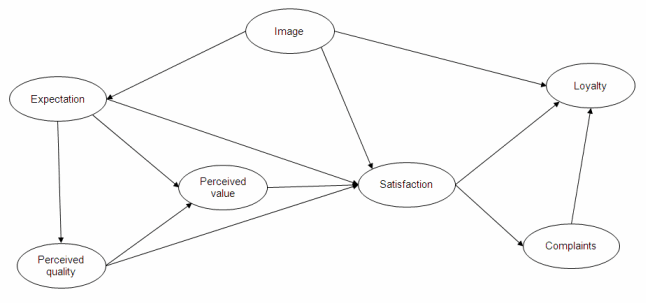Each latent variable is related to one or more manifest variables that are measured. In this application case, the manifest variables questions are on a 0-100 scale. For example, for the Image latent variable the five manifest variables are:

• It can be trusted in what it says and does
• It is stable and firmly established
• It has a social contribution for the society
• It is concerned with customers
• It is innovative and forward looking

## Dataset to create a basic XLSTAT-PLSPM project

An XLSTAT-PLSPM project sheet containing both the data and the results for use in this tutorial can be downloaded by clicking here. XLSTAT-PLSPM projects are special Excel workbook templates. When you create a new project, its default name starts with PLSPMBook. You can then save it to the name you want, but make sure you use the "Save" or "Save as" command of the XLSTAT-PLSPM toolbar to save it in the folder dedicated to the PLSPM projects using the *.ppm extension.

Note: when you open the PLSPathModeling_ECSI.ppm file, the graphical representation might look bad. This is due to the fact that the representation depends on your screen settings. To improve the display, click the "Optimize the display" button of the "Path modeling" toolbar (see below).

A raw XLSTAT-PLSPM project contains two sheets that cannot be removed:

• D1: This sheet is empty and you need to add all the input data that you want to use into that worksheet.
• PLSPMGraph: This sheet is blank and is used to design the model. When you select this sheet, the "Path modeling" toolbar is displayed. It is made invisible when you leave that sheet.

## Creating a basic XLSTAT-PLSPM project

To create the project used in this tutorial, we first generated a new project using the XLSTAT-PLSPM toolbar: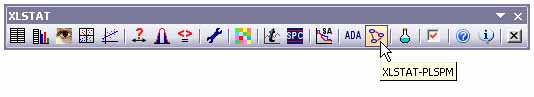PLS Path Modeling is a complex method and the PLSPM module of XLSTAT has many options and specificities. In order to simplify the application of a simple model, two displays are available.

The default one, called “classic”, displays the main functions necessary to apply PLS Path Modeling and a more sophisticated one, called “expert”, displays lots of new options like multigroup testing, moderating effect estimation, superbloc procedure… To modify this option, click on the “XLSTAT-PLPM options” button on the “XLSTAT-PLSPM” toolbar.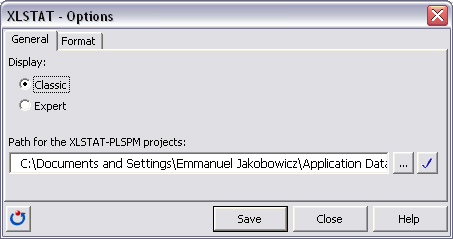We then saved it as PLSPathModeling_ECSI.ppm using the "Save as" command of the same toolbar.

Then, we copied the data that were available in an Excel file, and pasted them into the D1 sheet of the Project. Once this is done, you are ready to start creating the model. Move to the PLSPMGraph sheet. The "Path modeling" toolbar is displayed only on that sheet. You can find details on the function of each button in the help.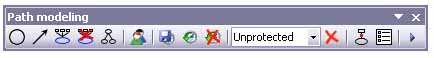To create several latent variables in a row, double click on the circle button so that it stays pressed while you add variables: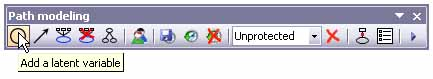You can then add the arrows that indicate how the latent variables are related. To create several arrows in a row, double click on the arrow button so that it stays pressed while you add the arrows.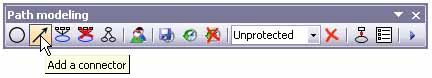To add an arrow, click on the latent variable from which it should start, then hold the left button of the mouse, then drag until the mouse cursor is over the latent variable where the arrow should end. Once an arrow is displayed you can still invert the direction or set it to double direction by using the contextual menu that you display clicking the right button of the mouse.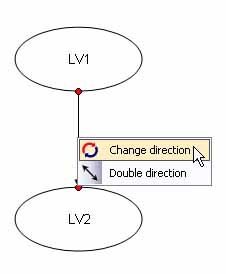Once all the arrows have been added, you can define the manifest variables that relate to each latent variable (this can also be done after adding the latent variables). To add manifest variables to a latent variable, the fastest way is to double-click the latent variable. This activates the D1 sheet and displays a dialog box where you give a proper name to the latent variable, select the manifest variables on D1 and define a few settings.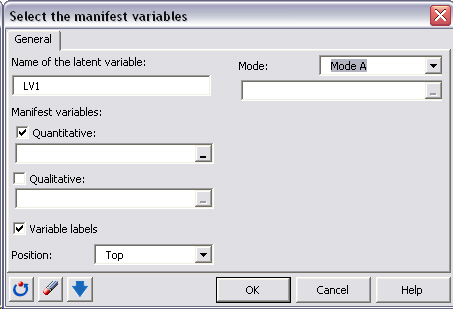The mode has to be defined. In Mode A (reflective mode) the latent variable is responsible for what is measured for the manifest variables, and in Mode B (formative mode), the manifest variables construct the latent variable.

For example, this is how the dialog box looked liked once filled in for the latent variable Expectation: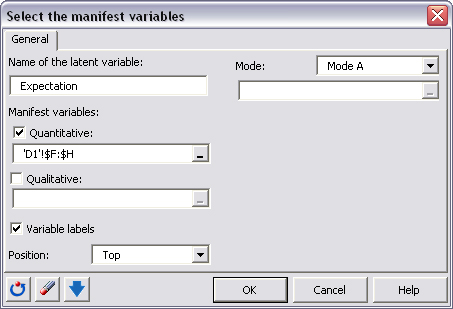The obtained model has the following form: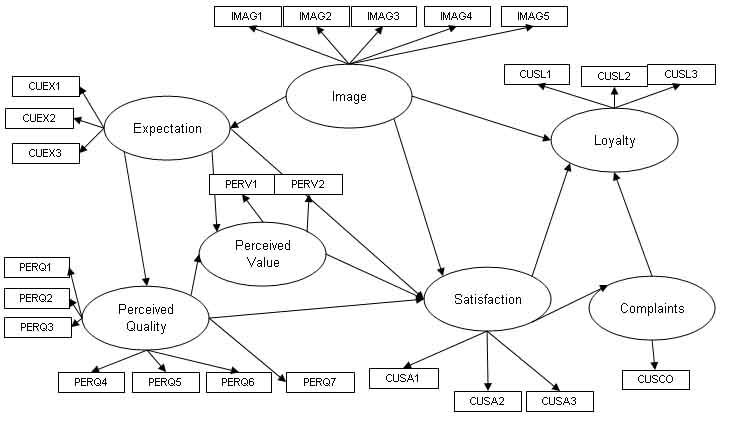Once the manifest variables have been defined for each latent variable and latent variables are linked, you can start computing the model. To run the model click the run button of the "Path modeling" toolbar.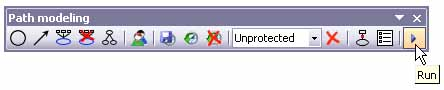This displays the "Run" dialog box, where many options are available. For this tutorial the following options have been used: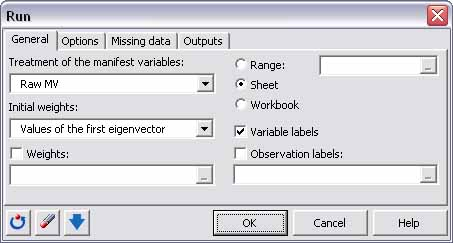PLS path modeling is based on an iterative algorithm and thus should be initialized. For this application, manifest variables (observed variables) are treated with no prior transformations (4 different settings are available) because all variables are on the same scale. The initial values for the outer weights are the values of the first eigenvector when performing a principal component analysis on the manifest variables associated to a latent variable (2 different settings are available).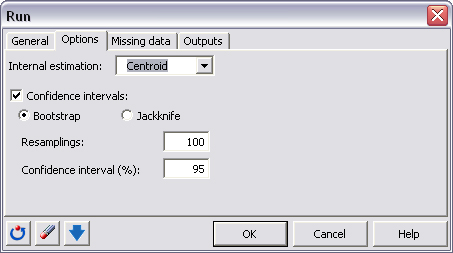We use the centroid scheme for inner weights estimation. Confidence intervals are obtained using bootstrap resample.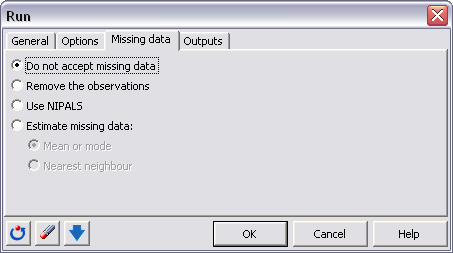In our simple example, there are no missing data in the dataset. We, thus, do not accept missing data.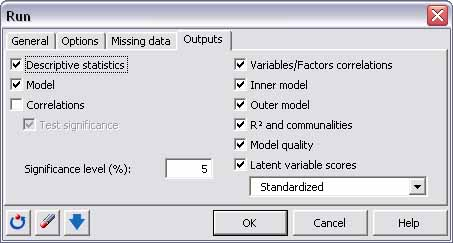Finally, for the output, all boxes are checked (except correlations) and we will study each output in the following part.

## Results and interpretation of a PLS-PM project

In the results, information related to the manifest variables, the measurement model and the structural model are first summarised.

The first important elements are the composite reliability indexes: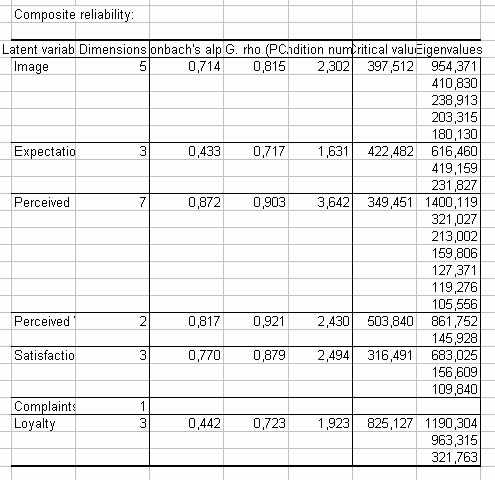In this application, latent variable are reflective. The blocks have to be one-dimensional. We can see that Dillon-Goldstein’s rho is higher than 0.7 and that the first eigenvalue is always far greater than the second one. Expectation and loyalty have bad values for the Cronbach’s alpha and a second dimension could be significant. In this tutorial, we will focus on the case of one dimension. If you are interested in further dimensions, you can study the correlations between manifest variables and factors in a principal component analysis applied on each block of manifest variables. We will not focus on that point and consider only one dimension.

Applying PLS path modelling gives the table with GoF indexes: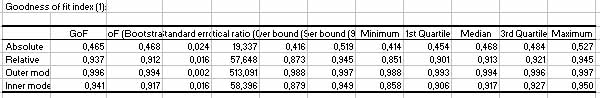We can see the absolute GoF is 0.465, very close to the bootstrap estimate. This value is hard to interpret; it could be useful when comparing the global quality of two groups of observations or two different models. The relative GoF is very high. So are inner and outer models GoF.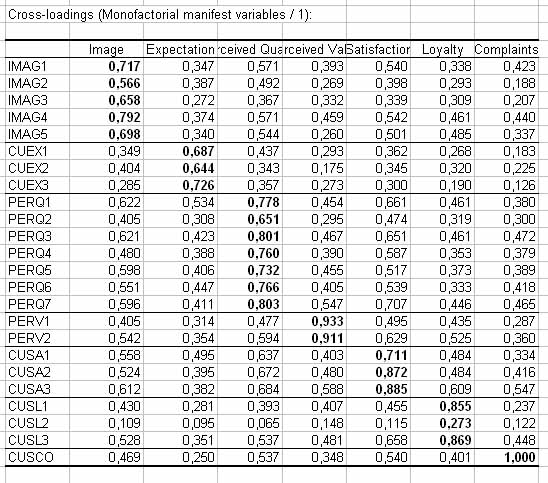In the case of our dataset, loadings between manifest variables and their own latent variable are the highest.

Then, outer weights and correlations are gathered in two large tables. If we study the correlations between manifest variables and latent variables: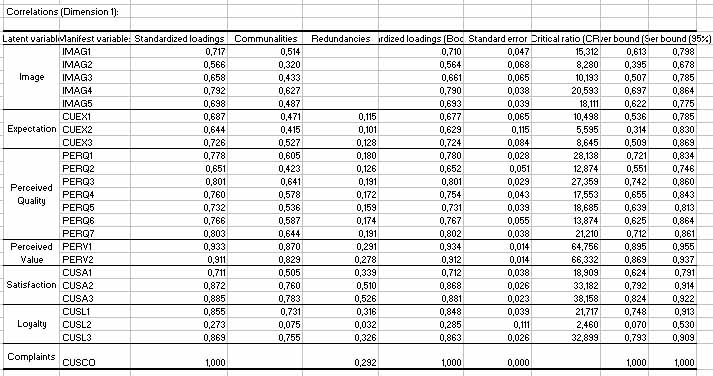We can see that, for example, the manifest variables CUSA3 and CUSA2 have a greater effect on satisfaction than CUSA1. These tables allow to see the impact of each manifest variables on its associated latent variable. The results associated to the structural model follow. For each latent variable, information on the structural model is gathered. In the case of satisfaction, we have: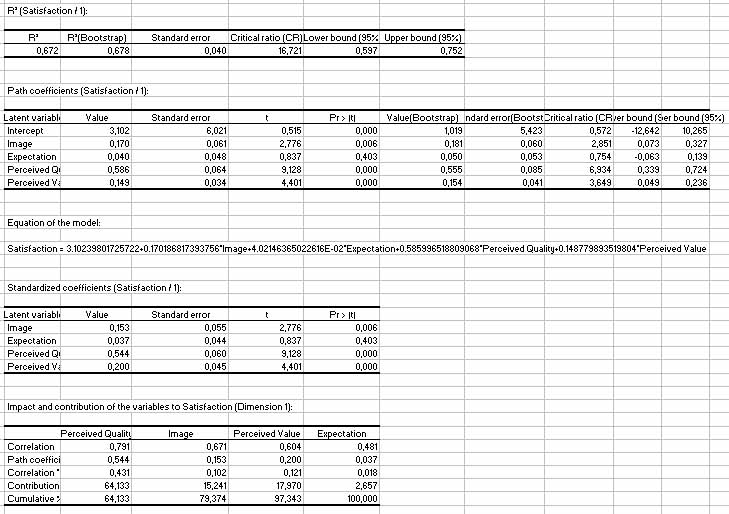A R’² of 0.672 can be considered as a good result. We can see that perceived quality has the greatest effect on satisfaction and that the impact of expectation is not significant. The last table summarizes the results and shows that perceived value contributes to 64% of the R’² of satisfaction. The chart illustrates these results: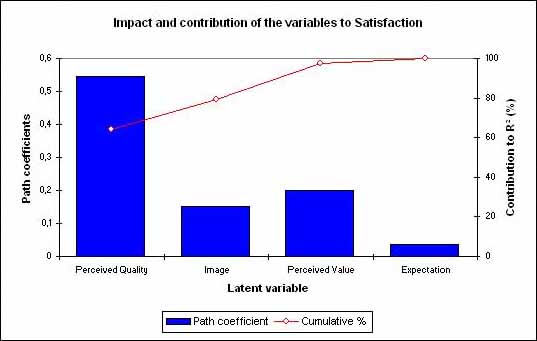The next table shows different predictive quality indexes associated to both outer and inner models for each latent variable. Mean values of these indices give a global quality value. We see that the mean of all R’² is 0.378 and the R’² of satisfaction is the highest one. Communalities are always greater that redundancies because PLSPM favours the measurement model in its estimation procedure.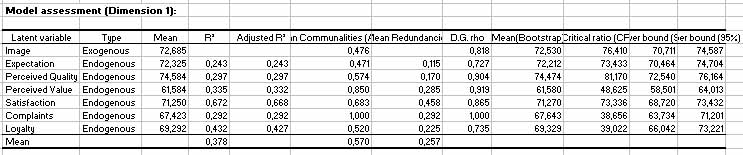One of the greatest advantages of PLSPM is the latent variable scores. They are given and can be used for other statistical treatments with XLSTAT. This study has shown how to use XLSTAT-PLSPM module in the case of real data. Once the model has been drawn, the procedure is simple. Once the model has been validated, interpretation of the result can be done by reading the tables with path coefficients and correlations.

### Graphical output of PLSPM

You can display many type of results on the path model with XLSTAT-PLSPM. Choose between al the possible indexes obtained when clicking on the button “Choose the results to display” in the “Path modelling” toolbar. The “results” dialog box appears. It has three pages:

• the first one concerns the display of indexes on the latent variables: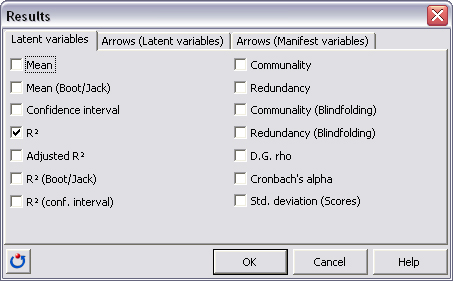• the second one concerns the display of coefficients and indexes on the arrows between latent variables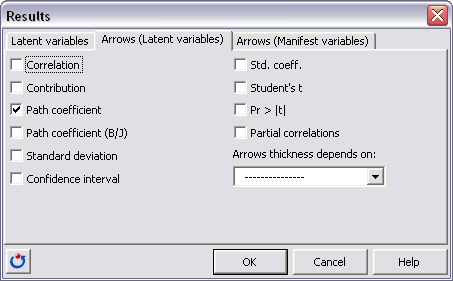• the third one concerns the display of coefficients and indexes on the arrows between manifest variables and latent variables: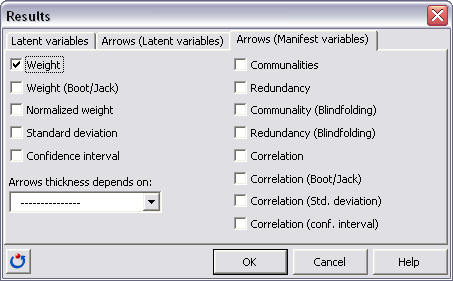Results appear on the path model in the worksheet PLSPMgraph when pushing the button “display result” on the “path modelling” toolbar. You can select the entire diagram and copy it to any other document.

#### Contact our technical support team: support@xlstat.com

https://cdn.desk.com/
false
desk